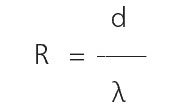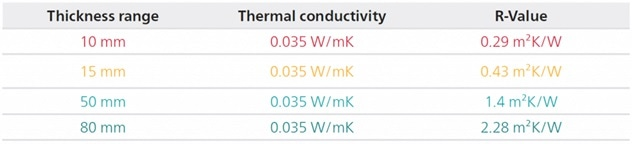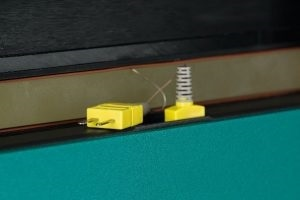# Measurement Range of a Heat Flow Meter

Are you responsible for improving the thermal transport properties of thermal insulation? Are you working in QA of insualtion materials and you collect thermal conductivity data all day long for CE-compliance? Are you working in R&D of polymers? Are you working for a certified laboratory or building material institute – and are you dealing with different kinds of materials? Are you dealing with one kind of material all day long? Are you dealing with thermal conductivity for specifiying the specsheet of your material? Then maybe the measurement range of a heat flow meter is very important for you?

## Thermal Resistance of Materials Governs Heat Flow Measurement Range

The question remains, although heat flow meters are used in various applications, is it the appropriate technique for measuring the thermal conductivity of any material or product?

The answer to this question is straightforward: The measurement range of heat flow meters is not defined in “thermal conductivity,” but in “thermal resistance”!

The ISO 8301 standard for heat flow meter technique clearly states the thermal resistance ranges that can be measured. Moreover, it is very easy to perceive. A stable heat flow, which is the most important for a heat flow meter result, can be achieved by forming a specific kind of thermal resistance to maintain a temperature gradient between the hot and the cold plate. This is the main reason for the usage of heat flow meters for insulating materials.

## Calculation of Thermal Resistance

The following equation can be used for calculating the thermal resistance:Equation 1. Calculation of thermal resistance

The ISO 8301 standard defines the measurement ranges as follows:

• Materials with a thermal resistance of 0.5 (m2K)/W or higher (up to 8 (m2K)/W) — mostly soft, low-conductive, and foamy material — can be measured without any challenges
• Samples of materials with thermal resistances between 0.3 and 0.5 (m2K)/W — slightly harder materials with low thermal conductivity — must be measured by using additional thermo-couples on the surfaces
• Samples of materials with thermal resistances between 0.1 and 0.3 (m2K)/W — materials with mostly harder surfaces and a higher thermal conductivity — require the use of additional thermocouples
• Materials with thermal resistances between 0.02 and 0.1 (m2K)/W can only be measured with additional thermocouples and results are less accurate
• Materials with thermal resistances lower than 0.02 (m2K)/W are out of the measurement range

The table below presents an example calculation based on glass wool that has a thermal conductivity of 0.035 W/m/K. It is evident that not all glass wool samples are appropriate for carrying out a proper measurement.Thermal resistances of glass wool in different thicknesses. Heat flow meter measurement range is defined by thermal resistance.

## What Can be Done if a Sample has a Lower Thermal Resistance than 0.5 (m2K)/W?

### For Low Conductive Insulating Materials

• The thickness of a sample should be increased by stacking the samples (for example, for thin XPS-Sheets with a thickness of 2 mm, place 10–15 sheets above one another. This makes thermal resistance 10–15 times higher and provides reliable results)
• The purported “blind specimen method” can be used, which involves placing an “unknown” sample to be measured between two samples of known thermal resistance. The thermal resistance of the “unknown” sample can be calculated from the total thermal resistance minus the thermal resistance of the known samples. The thermal conductivity of the sample can be calculated by knowing its thickness.

### For Higher Conductive Insulating Materials

• Additional thermocouples (= instrumentation kit) should be used to optimize the temperature measurement on the contact surfaces• Additional thermocouples enable the measurement of samples with a lower thermal resistance

## Conclusion

Heat flow meters are ideal instruments for measuring the thermal conductivity of a broad range of materials. In case researchers are not sure whether a heat flow meter can be used to measure the samples, it would be best to determine the thermal resistance of the samples.This information has been sourced, reviewed and adapted from materials provided by NETZSCH-Gerätebau GmbH.

## Citations

• APA

NETZSCH-Gerätebau GmbH. (2019, December 04). Measurement Range of a Heat Flow Meter. AZoM. Retrieved on August 10, 2020 from https://www.azom.com/article.aspx?ArticleID=16496.

• MLA

NETZSCH-Gerätebau GmbH. "Measurement Range of a Heat Flow Meter". AZoM. 10 August 2020. <https://www.azom.com/article.aspx?ArticleID=16496>.

• Chicago

NETZSCH-Gerätebau GmbH. "Measurement Range of a Heat Flow Meter". AZoM. https://www.azom.com/article.aspx?ArticleID=16496. (accessed August 10, 2020).

• Harvard

NETZSCH-Gerätebau GmbH. 2019. Measurement Range of a Heat Flow Meter. AZoM, viewed 10 August 2020, https://www.azom.com/article.aspx?ArticleID=16496.